Chemical Kinetics(20-11-2018)

# Chemical Kinetics(20-11-2018)

Test Description

## 30 Questions MCQ Test AAKSC Chemistry Mock test Series | Chemical Kinetics(20-11-2018)

Chemical Kinetics(20-11-2018) for Class 12 2022 is part of AAKSC Chemistry Mock test Series preparation. The Chemical Kinetics(20-11-2018) questions and answers have been prepared according to the Class 12 exam syllabus.The Chemical Kinetics(20-11-2018) MCQs are made for Class 12 2022 Exam. Find important definitions, questions, notes, meanings, examples, exercises, MCQs and online tests for Chemical Kinetics(20-11-2018) below.
Solutions of Chemical Kinetics(20-11-2018) questions in English are available as part of our AAKSC Chemistry Mock test Series for Class 12 & Chemical Kinetics(20-11-2018) solutions in Hindi for AAKSC Chemistry Mock test Series course. Download more important topics, notes, lectures and mock test series for Class 12 Exam by signing up for free. Attempt Chemical Kinetics(20-11-2018) | 30 questions in 45 minutes | Mock test for Class 12 preparation | Free important questions MCQ to study AAKSC Chemistry Mock test Series for Class 12 Exam | Download free PDF with solutions
 1 Crore+ students have signed up on EduRev. Have you?
Chemical Kinetics(20-11-2018) - Question 1

### In the reaction:A + 2B→ 3C+D ,which of the following expression does not described change in the concentration of various species as a function of time?

Detailed Solution for Chemical Kinetics(20-11-2018) - Question 1

For the given reaction.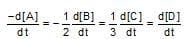Chemical Kinetics(20-11-2018) - Question 2

### The accompanying figure depicts the change in  concentration of species X and Y for the reaction , as a function of time. The point of intersection of the two curves represnts.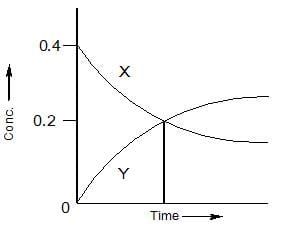Detailed Solution for Chemical Kinetics(20-11-2018) - Question 2

The intersection point indicates that half of the reactant X is converted into Y.

Chemical Kinetics(20-11-2018) - Question 3

### In a reaction, the rate expression is, rate = K [A] [B] 2/3 [C] 0, the order of reaction is:

Detailed Solution for Chemical Kinetics(20-11-2018) - Question 3

Order of reaction is sum of  powers raised on concentration terms in order to write rate expression.

Chemical Kinetics(20-11-2018) - Question 4

For a reversible reaction A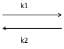B, Ist order in both the directions, the rate of reaction is given by:

Detailed Solution for Chemical Kinetics(20-11-2018) - Question 4

Rate  = K1[A]-K2[B] for a reversible reaction of 1 order opposed by 1 order.

Chemical Kinetics(20-11-2018) - Question 5

Which curve represnts Zero order reaction?

Detailed Solution for Chemical Kinetics(20-11-2018) - Question 5

For zero order reaction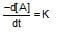On intergrating, -[A] = Kt + c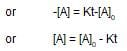or    y = c + mx

Chemical Kinetics(20-11-2018) - Question 6

For the decomposition of N2O5(g), it is given that: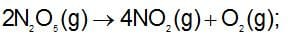Activation energy Ea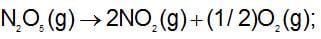Activation energy E'a

then:

Detailed Solution for Chemical Kinetics(20-11-2018) - Question 6

Energy of activation does not depend on the stoichiometry of change. It is characteristic value for a chemical      reaction.

Chemical Kinetics(20-11-2018) - Question 7

A reaction, occurs by the following mechanisum :

A⇆ A + A        .......(Slow)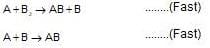Its order would be:

Detailed Solution for Chemical Kinetics(20-11-2018) - Question 7

rate = K[ A2]

Chemical Kinetics(20-11-2018) - Question 8

The rate of reaction becomes 2 times for every 100C rise in temperature. How the rate of reaction will increase when temperature is increased from 300C to 800C?

Detailed Solution for Chemical Kinetics(20-11-2018) - Question 8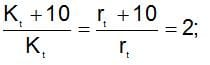rate2 =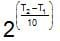x rate , the rate increases by 25 times, i.e., 32 times.

Chemical Kinetics(20-11-2018) - Question 9

A drop of solution (volume 0.05 mL) contains 3.0 x 10-6 mole of H+. If the rate constant of disappearance of H+ is 1.0 x 10-7 mol litre-1 sec-1. How long would it take for H+ in drop to disappear?

Detailed Solution for Chemical Kinetics(20-11-2018) - Question 9

Since rate constant = 1 x 10-7 mol litre-1 sec-1
∴   Zero order reaction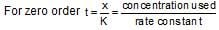0.05 mL has 3 x 10-6 mole of H+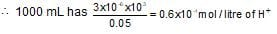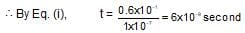Chemical Kinetics(20-11-2018) - Question 10

Ethylene is produced by cyclobutane as: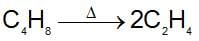The rate constant is 2.48 x 10-4 sec-1. In what time will the molar ratio of the ethylene to cyclobutane in reaction mixture attain the value equals to unity ?

Detailed Solution for Chemical Kinetics(20-11-2018) - Question 10Mole at t = 0    a    0

Mole at t = t    (a-x)    2x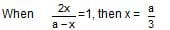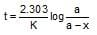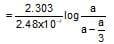=1635.2 second = 27.25 minute

Chemical Kinetics(20-11-2018) - Question 11

A reaction takes place in three steps with individual rate constant and activation energy.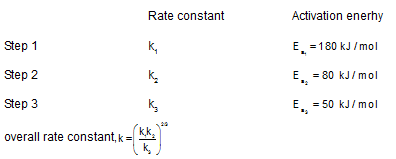Overall rate constant, K = (k1k3/k2)2/3

Overall activation energy of the reaction will be:

Detailed Solution for Chemical Kinetics(20-11-2018) - Question 11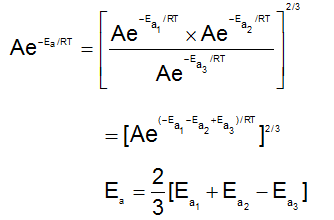= 2/3 [180 + 80 - 50] = 140KJ/mol

Chemical Kinetics(20-11-2018) - Question 12

In the following first order competing reactions :
A + Reagent ---> Product
B +  Reagent ---> Product
The ratio of K1/K2, if only 50% of B will have been reacted when 94% of A has been reacted in same time is:

Detailed Solution for Chemical Kinetics(20-11-2018) - Question 12

For 50% B reacted, K2 = (2.303/T2)log100/50

For 94% A reacted, K1 = (2.303/T1)log100/6

K2/K1 = t2/t1x0.3010/1.2218

Since t2 = t1, because 50% B has reacted when 94% A has reacted.

K2/K1 = 0.3010/1.2218 = 0.246 and K1/K2 = 4.06

Chemical Kinetics(20-11-2018) - Question 13

From the following data, the activation energy for the reaction (cal/mol):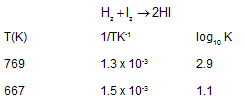Detailed Solution for Chemical Kinetics(20-11-2018) - Question 13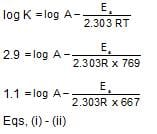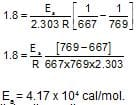Chemical Kinetics(20-11-2018) - Question 14

A substance undergoes first order decomposition. The decomposition follows two parallel first order reactions as: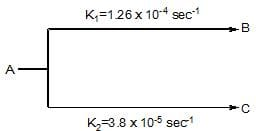​The percentage distribution of B and C are:

Detailed Solution for Chemical Kinetics(20-11-2018) - Question 14

For parallel path reaction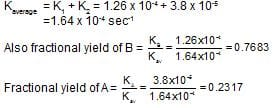Chemical Kinetics(20-11-2018) - Question 15

Mathematical expression for t1/4, i.e., when (1/4)th reaction is over following first order kinetics can be given by:

Detailed Solution for Chemical Kinetics(20-11-2018) - Question 15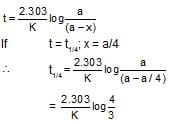Chemical Kinetics(20-11-2018) - Question 16

The rateconstant of a second order reaction is 10-2 (Ms)-1. The rate constant expressed in cc. molecule-1 min-1 is:

Detailed Solution for Chemical Kinetics(20-11-2018) - Question 16

K = 10-2 mol-1 litre sec-1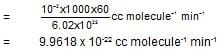Chemical Kinetics(20-11-2018) - Question 17

The rate of disappearance of SO2 in the reaction; 2SO2 + O2 ---> 2SO3 is 1.28 x 10-3 g/sec. The the rate of formation of SO3 is:

Detailed Solution for Chemical Kinetics(20-11-2018) - Question 17

The rate of formation of SO3 is 1.28×10−3g/sec.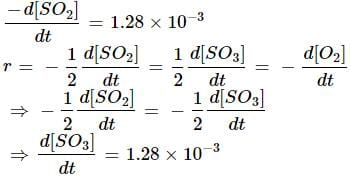Chemical Kinetics(20-11-2018) - Question 18

The rate of a first order reaction is 1.5 x 10-2 M min-1 at 0.5 M concentration of reactant. The half-life of reaction is:

Detailed Solution for Chemical Kinetics(20-11-2018) - Question 18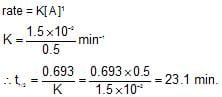Chemical Kinetics(20-11-2018) - Question 19

For a reaction aA -->bB when [A] = 2.2 mM, the rate was found to be 2.4 mMs-1. On reducing concentration of [A] to half , the rate changes to 0.6 mMs-1. The order of reaction with respect to A is:

Detailed Solution for Chemical Kinetics(20-11-2018) - Question 19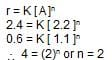Chemical Kinetics(20-11-2018) - Question 20

​During the kinetic study of the reaction  following results were obtained.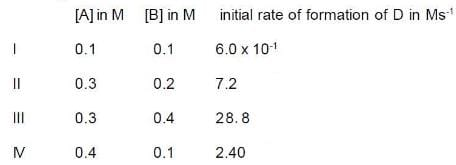On the basis the data, which one is correct?

Detailed Solution for Chemical Kinetics(20-11-2018) - Question 20

Use : r = K [ A ]m [ B ]n

Chemical Kinetics(20-11-2018) - Question 21

The reaction L ---> 4  is started with 10 g of L. After 30 and 90 mintue, 5 g and 1.25 g of L are left respectively. The order of reaction is :

Detailed Solution for Chemical Kinetics(20-11-2018) - Question 21

The given data suggests that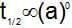which is obeyed by first order reaction.

Chemical Kinetics(20-11-2018) - Question 22

The differential rate expression for the reaction H2 + I--> 2HI is:

Detailed Solution for Chemical Kinetics(20-11-2018) - Question 22

follow review of rate of reaction.

Chemical Kinetics(20-11-2018) - Question 23

In a first order reaction the concentration of reactant decreases from 800 mol/dm3 to 50 mol/dm3 in 2 x 104 sec. The rate constant of reaction in sec-1 is:

Detailed Solution for Chemical Kinetics(20-11-2018) - Question 23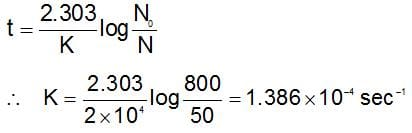Chemical Kinetics(20-11-2018) - Question 24

Plots showing the variation of the rate constant (k) with temperature (T) are given below. The plot that follows Arrhenius equation is:

Detailed Solution for Chemical Kinetics(20-11-2018) - Question 24

i.e , K =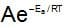increase exponentially, with rise in temperature.

Chemical Kinetics(20-11-2018) - Question 25

The rate constants of three reactions involving reactant A only obeying I, II, III order respectively is same. Which of the following is true?​

Detailed Solution for Chemical Kinetics(20-11-2018) - Question 25

r1 = K [ A ]1 ; r2 = K [ A ]2 ; r3 = K [ A ]3

Chemical Kinetics(20-11-2018) - Question 26

loge K vs. 1/T plots shows a straight line having slope of 1200 and intercepts on the Y-axis of 2, then :

Detailed Solution for Chemical Kinetics(20-11-2018) - Question 26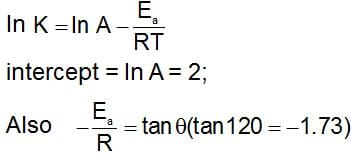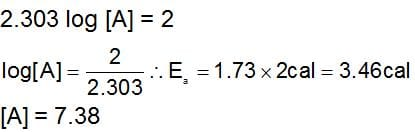Chemical Kinetics(20-11-2018) - Question 27

A reaction occurs in three rate detemining steps having rate constant K1, K2 and K3 respectively and arrehenius factor A1, A2, A3 respectively. The overall rate constant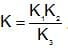. If energy of activations for each step is Ea1, Ea2, and Ea3 respectively, then overall energy of activation is:

Detailed Solution for Chemical Kinetics(20-11-2018) - Question 27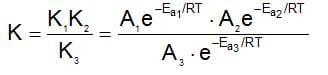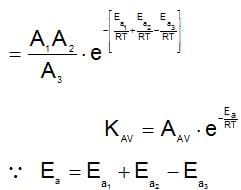Chemical Kinetics(20-11-2018) - Question 28

At 3 km altitude, water boils at 900C and 300 seconds are taken to cook a ‘3 minute egg’. The temperature coefficient for the process of cooking is:

Detailed Solution for Chemical Kinetics(20-11-2018) - Question 28

Temperature coefficient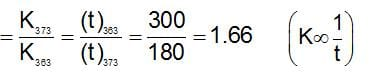Chemical Kinetics(20-11-2018) - Question 29

Select the incorrect statement:

Detailed Solution for Chemical Kinetics(20-11-2018) - Question 29

According to Le Chatelier’s principle rate of exothermic reactions decreases with an increase in temperature.

Chemical Kinetics(20-11-2018) - Question 30

The rate expression for a reaction is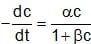(α and β > 0). The half life of the reaction can be given by:

Detailed Solution for Chemical Kinetics(20-11-2018) - Question 30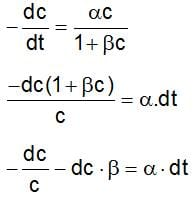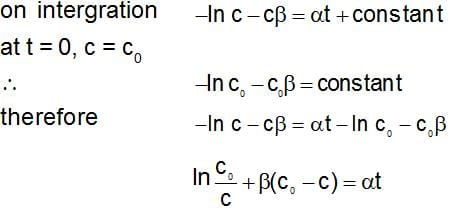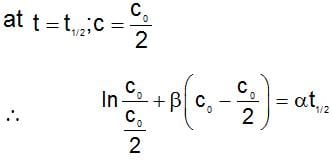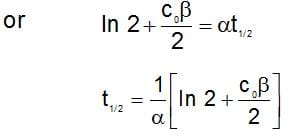## AAKSC Chemistry Mock test Series

1 docs|26 tests
 Use Code STAYHOME200 and get INR 200 additional OFF Use Coupon Code